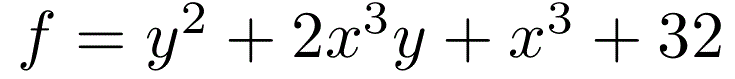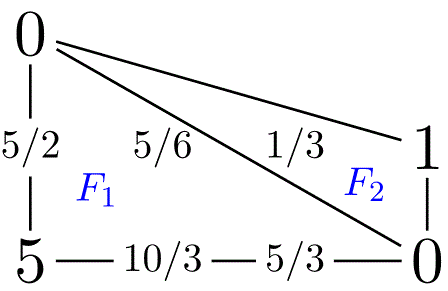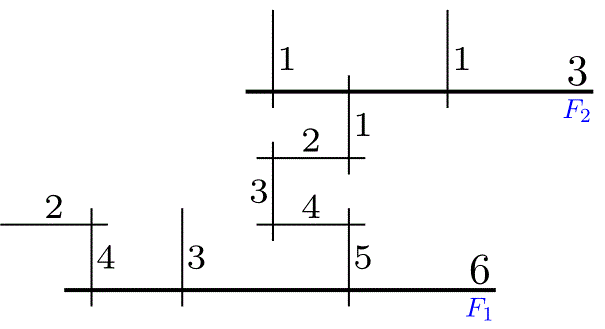# Models of curves

### by Tim Dokchitser

This is a Magma script to compute regular models of 'generic' curves over discrete valuation rings. Assumes the curve is given by one affine equation f(x,y)=0, for example elliptic, hyperelliptic, plane etc. The script is based on the paper Models of curves over DVRs.

→  one zip archive  ←

Individual files:

test.m - this is the script to execute
texmodel.m - main library used by the script
test.tex - TeX file, compiled by test.m

Assuming you have Magma and pdflatex on your system, executing test.m should compute the models specified in the file, write them to test.inc, and compile test.tex (that includes test.inc) to test.pdf automatically. It uses standard latex packages pbox and tkz-graph.→→For the notation in the pictures, see Table 1 on p.7 of the paper.